# Clairaut's equation

## Definition

### Form of the differential equation

Clairaut's equation is a first-order differential equation of the form: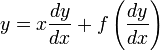$y = x \frac{dy}{dx} + f\left(\frac{dy}{dx}\right)$

Here,$f$ is a suitable function.

### Solution method and formula

Differentiate both sides with respect to$x$ and obtain:$\frac{dy}{dx} = \frac{dy}{dx} + \left[x + f'\left(\frac{dy}{dx}\right)\right]\frac{d^2y}{dx^2}$

Cancel the common term from both sides and obtain:$0 = \left[x + f'\left(\frac{dy}{dx}\right)\right]\frac{d^2y}{dx^2}$

This gives two possible solution types: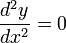$\frac{d^2y}{dx^2} = 0$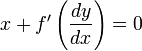$x + f'\left(\frac{dy}{dx}\right) = 0$

Note that when we differentiated, we lost information, so it is not true that all solutions of the differentiated equation solve the original equation. Rather, we need to plug these solutions into the original equation to constrain them. We have the following:

• The solutions for the$\frac{d^2y}{dx^2} = 0$ case are straight lines of the form$y = Cx + f(C)$. This is the solution family for the general solution.
• The solution for the$x + f'\left(\frac{dy}{dx}\right) = 0$ case is unique: it is a parametric curve given by$x = -f'(p), y = f(p) - pf'(p)$ where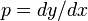$p = dy/dx$ is the parameter moving along the curve. This is the singular solution. The curve representing this is the envelope of the general solution curves. In order to verify that this solution is correct, we can calculate$dy/dx$ using parametric differentiation with respect to$p$ and check that it is indeed equal to$p$.

## Related equations

• Lagrange equation is a more general setup that includes Clairaut's equation as a special case in terms of method, albeit a special case that is qualitatively somewhat different.

## Examples

We will denote$dy/dx$ by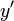$y'$.

### Simple example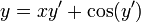$y = xy' + \cos(y')$

The solutions are:

• The solution family for the general solution is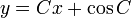$y = Cx + \cos C$, with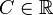$C \in \R$.
• There is a special solution given parametrically by$x = \sin p, y = \cos p + p \sin p$, with$p \in \R$. Note that this is a single solution; the parameter$p$ varies to cover the points of the solution, and it is different from the notion of parameter for a family of solutions.

### Clairaut's after substitution

Consider the equation:$0 = x^2y' + \cos(y + xy')$

We first note that the expression whose cosine is being taken is the derivative of$xy$, hence the natural choice of substitution is to try for$u = xy$. Our next step is to try and write$x^2y'$ in terms of$u$ and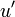$u'$. Recall that$u = xy$ and$u' = y + xy'$, so$xy' = u' - y$, so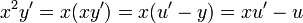$x^2y' = x(xy') = x(u' - y) = xu' - u$, so:$0 = xu' - u + \cos(u')$

Rearrange to get: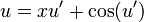$u = xu' + \cos(u')$

This is a Clairaut's equation with dependent variable$u$ and independent variable$x$, so the solutions are:

• The solution family for the general solution is$xy = Cx + \cos C$, with$C \in \R$. This can be rewritten as$y = C + (\cos C)/x$, with$C \in \R$.
• There is a special solution given parametrically by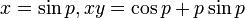$x = \sin p, xy = \cos p + p \sin p$, with$p \in \R$. This can be rewritten as$x = \sin p, y = \cot p + p$, with$p \in \R$.